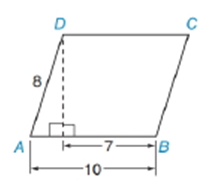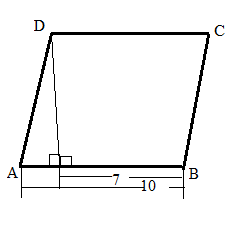Chapter 11.2, Problem 39EElementary Geometry For College St...

7th Edition
Alexander + 2 others
ISBN: 9781337614085

Solutions

Chapter
SectionElementary Geometry For College St...

7th Edition
Alexander + 2 others
ISBN: 9781337614085
Textbook Problem

In parallelogram ABCD, find to the nearest degree:a) m ∠ A b) m ∠ BTo determine

a) To find:

To find mA by using the following figure,Explanation

Consider the following figure,

In parallelogram ABCD let us take angle at A is α

General formula for cosine ratio and sin ratio is given below,

From the above figure shows that AD = 8 is the hypotenuse value since it is opposite to right angle

To determine

b) To find:

To find mB by using the following figure,Still sussing out bartleby?

Check out a sample textbook solution.

See a sample solution

The Solution to Your Study Problems

Bartleby provides explanations to thousands of textbook problems written by our experts, many with advanced degrees!

Get Started

Find more solutions based on key concepts